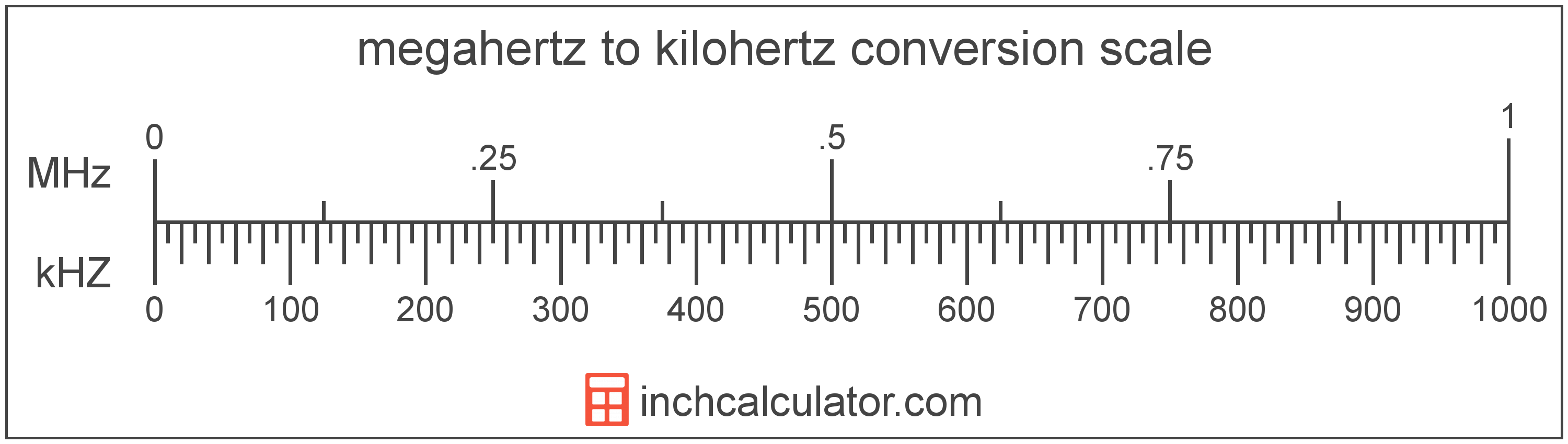# Megahertz to Kilohertz Conversion

Enter the frequency in megahertz below to get the value converted to kilohertz.

Results in Kilohertz:1 MHz = 1,000 kHZ

## How to Convert Megahertz to KilohertzTo convert a megahertz measurement to a kilohertz measurement, multiply the frequency by the conversion ratio.

Since one megahertz is equal to 1,000 kilohertz, you can use this simple formula to convert:

kilohertz = megahertz × 1,000

The frequency in kilohertz is equal to the megahertz multiplied by 1,000.

For example, here's how to convert 5 megahertz to kilohertz using the formula above.
5 MHz = (5 × 1,000) = 5,000 kHZ

### How Many Kilohertz are in a Megahertz?

There are 1,000 kilohertz in a megahertz, which is why we use this value in the formula above.

1 MHz = 1,000 kHZ

## Megahertz

Megahertz is a measure of frequency equal to one million cycles per second.

The megahertz is a multiple of the hertz, which is the SI derived unit for frequency. In the metric system, "mega" is the prefix for 106. Megahertz can be abbreviated as MHz; for example, 1 megahertz can be written as 1 MHz.

## Kilohertz

Kilohertz is a measure of frequency equal to one thousand cycles per second.

The kilohertz is a multiple of the hertz, which is the SI derived unit for frequency. In the metric system, "kilo" is the prefix for 103. Kilohertz can be abbreviated as kHZ; for example, 1 kilohertz can be written as 1 kHZ.

## Megahertz to Kilohertz Conversion Table

Megahertz measurements converted to kilohertz
Megahertz Kilohertz
0.001 MHz 1 kHZ
0.002 MHz 2 kHZ
0.003 MHz 3 kHZ
0.004 MHz 4 kHZ
0.005 MHz 5 kHZ
0.006 MHz 6 kHZ
0.007 MHz 7 kHZ
0.008 MHz 8 kHZ
0.009 MHz 9 kHZ
0.01 MHz 10 kHZ
0.02 MHz 20 kHZ
0.03 MHz 30 kHZ
0.04 MHz 40 kHZ
0.05 MHz 50 kHZ
0.06 MHz 60 kHZ
0.07 MHz 70 kHZ
0.08 MHz 80 kHZ
0.09 MHz 90 kHZ
0.1 MHz 100 kHZ
0.2 MHz 200 kHZ
0.3 MHz 300 kHZ
0.4 MHz 400 kHZ
0.5 MHz 500 kHZ
0.6 MHz 600 kHZ
0.7 MHz 700 kHZ
0.8 MHz 800 kHZ
0.9 MHz 900 kHZ
1 MHz 1,000 kHZ